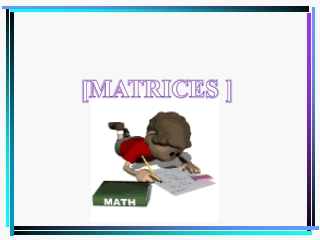DownloadDownload Presentation[MATRICES ]

# [MATRICES ]

Télécharger la présentation## [MATRICES ]

- - - - - - - - - - - - - - - - - - - - - - - - - - - E N D - - - - - - - - - - - - - - - - - - - - - - - - - - -
##### Presentation Transcript

1. [MATRICES ]

2. Matrix (plural: matrices) • Matrix • a rectangular array of numbers written within brackets • Represented with a capital letter and classify by its dimension • Dimensions of a Matrix/Order of a Matrix • determine by the number of horizontal rows and the number of vertical columns • Matrix Element • each number in a matrix

3. Writing the dimensions of a matrix. 4 columns A= 3 rows Matrix A is a 3 x 4 matrix.

4. 6 5 • -3 -7 1 0 9 -4 1/3 -3 10 0 1 -5 -6.2 9 • 5 0 -2 0.5 17 Write the dimensions or order of each matrix. 1. 3. 2. 4. 1 X 3 3 X 3 3 X 2 2 X 3

5. Identifying a Matrix Element aij denotes the element of the matrix A on the ithrow and jthcolumn. Example: Identify element a13in Matrix A. Answer: a13 means the element in row 1, column 3. a13 = 1

6. Identify each matrix element • a33 • a11 • a21 • a34 • a23 A= • a33 = 4 • a11 = -5 • a21 = 3 • a34 = 2 • a23 = -9

7. Adding and Subtracting Matrices • to add or subtract matrices A and B with the same dimensions, add or subtract the corresponding elements ***Note: you can only add or subtract matrices with the same dimensions.

8. Properties: Matrix Addition If A, B, and C are m x n matrices, then a. Closure Property A + B is an m x n matrix • Commutative Property A + B = B + A • Associative Property for Addition (A + B) + C = A + (B + C) • Additive Identity Property There exist a unique m x n matrix O such that O + A = A + O = A • Additive Inverse Property For each A, there exists a unique opposite –A. A + (-A) = O

9. -4 3 0 6 5 10 • 9 -3 -9 6 12 -3 1 2 -4 3. 4. 1. • -2 0 3 -5 7 -3 5 -1 10 • -9 7 -2 1 8 • -12 7 -8 -4 -2 0 4 -3 14 4 7 -3 -6 1 19 - + - = = = -3 1 2 -4 -1 5 2. -12 24 -3 5 -1 10 -15 25 -1 1 -2 15 + = Find the sum or difference of each matrix. 1.

10. -14 -5 0 2 1. • 5 0 -2 , • -10 5 0 -2 3 1. -1 10 -5 0 2 -3 => Identify whether the two matrices are additive inverse or not. Yes. Find the additive inverse of the given matrix.

11. Solving Matrix Equations • Matrix Equation • an equation in which the variable is a matrix • Equal Matrices • matrices with the same dimensions and with equal corresponding elements

12. 0 1 8 9 0 1 8 9 • 1 3 2 • 1 3 2 = = X - X - • 1 3 2 0 1 8 9 X = + 1 2 11 11 X = Solving a Matrix Equation • Solve for the matrix X. • Solution:

13. 10 7 -4 4 -1 0 2 5 = X + • 7 -6 -1 Answer: X = 2 1 -1 0 2 1 11 3 -13 15 -9 8 - X = -9 -2 12 -15 -11 -7 Answer: X = Do these… Solve for Matrix X. 1. 2.

14. -8/4 6 – 3 15/3 4 - 4 -2 3 5 0 , Determining Equal Matrices Determine whether the two matrices in each pair are equal. 1. 2. 4 6 8 , 8/2 18/3 16/2 No, because they do not have the same dimensions. Yes, because they have the same dimensions and the corresponding elements are equal.

15. x + 8 -5 3 -y 38 -5 3 4y – 10 = Finding Unknown Matrix Elements • Solve the equation for x and y. Solution: x + 8 = 38 x = 30 -y = 4y – 10 -5y = -10 y = 2

16. , -9 x + y 3x 4 Do these… Solve each unknown variable in each equation. 1. 2. x = -3, y = 7 • 4 8 12 4x – 6 -10t + 5x 4x 15t +1.5x = x = 2; t = 3/5